# Conversions

Conversion functions to/from non SI units and type definitions of non SI units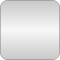# Package Contents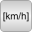NonSIunits Type definitions of non SI units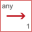to_unit1 Change the unit of a Real number to unit="1"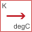to_degC Convert from Kelvin to degCelsius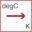from_degC Convert from degCelsius to Kelvin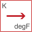to_degF Convert from Kelvin to degFahrenheit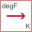from_degF Convert from degFahrenheit to Kelvin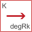to_degRk Convert from Kelvin to degRankine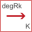from_degRk Convert from degRankine to Kelvin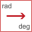to_deg Convert from radian to degree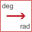from_deg Convert from degree to radian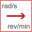to_rpm Convert from radian per second to revolutions per minute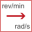from_rpm Convert from revolutions per minute to radian per second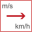to_kmh Convert from metre per second to kilometre per hour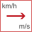from_kmh Convert from kilometre per hour to metre per second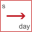to_day Convert from second to day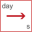from_day Convert from day to second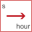to_hour Convert from second to hour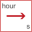from_hour Convert from hour to second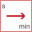to_minute Convert from second to minute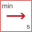from_minute Convert from minute to second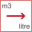to_litre Convert from cubic metre to litre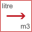from_litre Convert from litre to cubic metre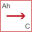from_Ah Convert from Ampere hours to Coulomb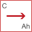to_Ah Convert from Coulomb to Ampere hours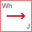from_Wh Convert from Watt hour to Joule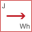to_Wh Convert from Joule to Watt hour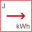to_kWh Convert from Joule to kilo Watt hour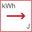from_kWh Convert from kilo Watt hour to Joule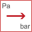to_bar Convert from Pascal to bar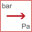from_bar Convert from bar to Pascal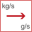to_gps Convert from kilogram per second to gram per second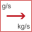from_gps Convert from gram per second to kilogram per second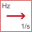from_Hz Convert from Hz to rad/s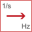to_Hz Convert from rad/s to Hz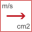to_cm2 Convert from square metre to square centimetre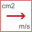from_cm2 Convert from square centimetre to square metre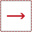ConversionIcon This icon will be removed in future Modelica versions.

# Information

This information is part of the Modelica Standard Library maintained by the Modelica Association.

This package provides conversion functions from the non SI Units defined in package Modelica.SIunits.Conversions.NonSIunits to the corresponding SI Units defined in package Modelica.SIunits and vice versa. It is recommended to use these functions in the following way (note, that all functions have one Real input and one Real output argument):

```  import SI = Modelica.SIunits;
import Modelica.SIunits.Conversions.*;
...
parameter SI.Temperature     T   = from_degC(25);   // convert 25 degree Celsius to Kelvin
parameter SI.Angle           phi = from_deg(180);   // convert 180 degree to radian
parameter SI.AngularVelocity w   = from_rpm(3600);  // convert 3600 revolutions per minutes